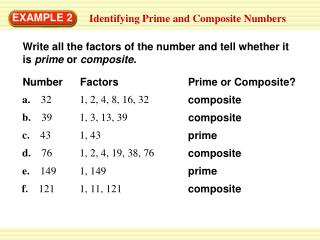DownloadDownload PresentationEXAMPLE 2

# EXAMPLE 2

Download Presentation## EXAMPLE 2

- - - - - - - - - - - - - - - - - - - - - - - - - - - E N D - - - - - - - - - - - - - - - - - - - - - - - - - - -
##### Presentation Transcript

1. Number Factors Prime or Composite? EXAMPLE 2 Identifying Prime and Composite Numbers Write all the factors of the number and tell whether it is prime or composite. a. 32 1, 2, 4, 8, 16, 32 composite b. 39 1, 3, 13, 39 composite c. 43 1, 43 prime d. 76 1, 2, 4, 19, 38, 76 composite e. 149 1, 149 prime composite f. 121 1, 11, 121

2. So, 450 = 2 3 3 5 5. EXAMPLE 3 Writing Prime Factorization Write the prime factorization of 450. Three factor trees are shown. Notice that each factor tree produces the same prime factorization, differing only in the order of the factors.

3. ANSWER Using exponents, the prime factorization of 450 is 2• 32•52 EXAMPLE 3 Writing Prime Factorization

4. Tell whether the number is prime or composite. If it is composite, write its prime factorization using exponents. Using exponents, the prime factorization of 24 is 23•3 6. 24 ANSWER Composite; It has positive factors other then 1 and itself. for Examples 2 and 3 GUIDED PRACTICE

5. Tell whether the number is prime or composite. If it is composite, write its prime factorization using exponents. Using exponents, the prime factorization of 51 is 3 • 17 7. 51 ANSWER Composite; It has positive factors other then 1 and itself. for Examples 2 and 3 GUIDED PRACTICE

6. Tell whether the number is prime or composite. If it is composite, write its prime factorization using exponents. 8. 73 ANSWER Prime; only positive factors are 1 and itself. for Examples 2 and 3 GUIDED PRACTICE

7. Tell whether the number is prime or composite. If it is composite, write its prime factorization using exponents. Using exponents, the prime factorization of 560 is 24• 5 • 7 9. 560 ANSWER Composite; It has positive factors other then 1 and itself. for Examples 2 and 3 GUIDED PRACTICE